# (Answers) Class 10 Chemical Reactions and Equations Test – 1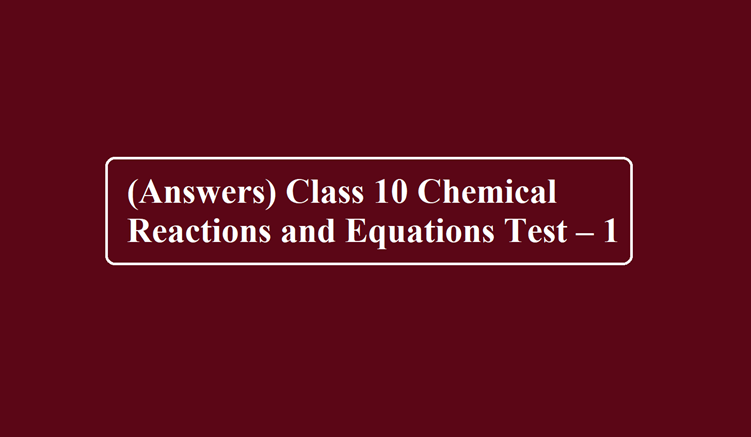# (Answers) Class 10 Chemical Reactions and Equations Test – 1

(Answers) Class 10 Chemical Reactions and Equations

Ans 1. The word equation for the reaction is:
Iron + Water → Ferric oxide + Hydrogen
The symbol equation for the same reaction is:
Fe + H2O → Fe2O3 + H2 ­
The balancing of the equation is done in the following steps:
Step I. Let us count the number of atoms of all the elements in the reactants and products on
both sides of the equation.

 Element No. of atoms of reactants (L.H.S.) No. of atoms of products (R.H.S.) Fe 1 2 H 2 2 O 1 3

From the equation, we can say that the number of H atoms is equal on both sides. At the same
time, the number of Fe and O atoms are not equal.
Step II. On inspection, the number of O atoms in the reactant (H2O) is 1 while in the product (Fe2O3), these are 3. To balance the atoms, put co-efficient 3 before H2O on the reactant’s side. The partially balanced equation may be written as:
Fe + 3H2O → Fe2O3 + H2 ­
Step III. To equate H atoms, put coefficient 3 before H2 on the product side. As a result, the H atoms on both sides of the equation become 6 and are thus balanced. The partially balanced equation may now be written as:
Fe + 3H2O → Fe2O3 + 3H2 ­
Step IV. To balance the Fe atoms, put coefficient 2 before Fe on the reactant side. The equation formed may be written as:
2Fe + 3H2O → Fe2O3 + 3H2 ­
Step V. On final inspection, the number of atoms of all the elements on both sides of the equation is equal. Therefore, the equation is a balanced equation.

(ii) When potassium chlorate (KClO3) is heated in the presence of a manganese dioxide catalyst,
it decomposes to form potassium chloride and oxygen gas. This can be written as: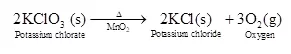Ans 2. (i) Carbon monoxide upon reaction with oxygen produces carbon dioxide
CO (g) + O2 (g) → CO2 (g)
(ii) When ferrous sulphate is heated strongly it is an example of a decomposition reaction. Ferrous
sulphate decomposes to form a ferric oxide, sulphur dioxide and sulphur trioxide: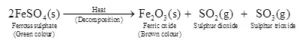Ans 3. The reaction involved is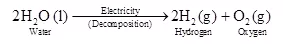In this reaction, a single compound i.e water splits up into two simpler substances, hydrogen, and oxygen by the action of electricity. So, this is a decomposition reaction. It is also called the electrolysis of water.

Ans 4. As light consists of photons (discrete packets of energy) which are the source of energy. They cause the decomposition of silver chloride. Similarly, silver bromide also undergoes decomposition in the presence of sunlight.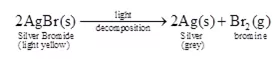Generally, silver salts are very much sensitive to light, so they decompose easily. This property forms the basis of black and white photography.

Ans 5. BaCl2 (aq) + Na2SO4 (aq) → NaCl (aq) + BaSO4 (aq)

Ans 6. The reaction involved is
H2S + Cl2 → S + 2 HCl
In this reaction, H2S is changing into S. That is, hydrogen is being removed from hydrogen Sulphide, and hydrogen sulphide is being oxidized to sulpher. In this reaction, Cl2 is changes into HCl. That is, hydrogen is being added to chlorine. hence chlorine is being reduced to hydrogen chloride. The oxidation-reduction reaction between hydrogen sulphide and chlorine can be shown as: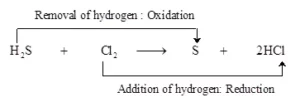Substance oxidised = Reducing agent = H2S
Substance reduced = Oxidising agent = Cl2

Ans 7. In the presence of moisture rusting of iron takes place
4 Fe + 3 O2 + 2 xH2O → 2 Fe2O3.xH2O

Ans 8. (i) The burning of natural gas is an exothermic reaction because heat is produced in this reaction
CH4 (g)     +      2 O2 (g)     →     CO2 (g)     +      H2O (l)
Methane          Oxygen           Carbon Dioxide      Water

(ii) During respiration, glucose combines with oxygen in the cells of our body to form carbon dioxide and water along with the production of energy.
6H12O6     +     O2        →       CO2        +        2 H2O      +       Energy
Glucose           Oxygen           Carbon Dioxide

(iii) The decomposition of calcium carbonate is an endothermic reaction because heat energy is absorbed in this reaction.
CaCO3 → CaO + CO2

(iv) This is because sunlight energy is absorbed during the process of photosynthesis by green plants.
6CO2(aq) + 6H2O (l) → C6H12O6(aq) + 6O­2 (aq)

(v) The main objective of flushing Nitrogen into food packets containing oil and fat products is to avoid rancidity, which results when the oil or fat combines with oxygen and produces an unpleasant odour and taste. As a result, flushing Nitrogen creates an unreactive environment, which prevents rancidity.

Ans 9. Cu + Ag NO3 → Cu(NO3)2

Ans 10. Mg + Cl2 → MgCl2
Cl2 Undergo reduction

Ans 11. (C) Loss of electrons & gain of electrons respectively

Ans 12. (C) Attack of H2O, O2 & CO2 present in the atmosphere on the surface of copper.

Ans 13. Here the addition of oxygen to carbon forms CO so it is the Oxidation of Carbon and thus carbon is a reducing agent.

Ans 14. (B) Oxidizing agent

Ans 15. (C) Reduction is gain of electron

Ans 16. (B) Nitrogen is unreactive gas and there is no oxygen in the air to cause its oxidation.

Ans 17. (A) Exothermic reaction

Ans 18.  All of the above.

Ans 19. Zinc is oxidised to give ZnSO4 which also means loss of electrons.

Ans 20. (A) An endothermic reaction

Ans 21. (C) Exothermic reaction

Ans 22. (C) donate electron

Ans 23. (A) Iron gets oxidised

Ans 24. (B)
2AlCl3   +   3Ca(OH)2  → 2Al(OH)3  +  3CaCl2

Ans 25. (B)
CH4   +   O2  →  CO2   +   H2O
Here CH4 gas gets oxidized while oxygen gas gets reduced

(Answers) Class 10 Chemical Reactions and Equations

Class 10 Chemical Reactions and Equations Test – 1